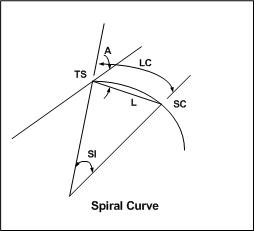* Log In to use the Calculate function * Become a Member!

Calculate The Deflection Angle For Spiral Curve:

Calculation:
Designer/Checker:

Input:Average degree of spiral (SI - deg)
Length of spiral (LS - ft or m)
Chord Length (L - ft or m)

Output: